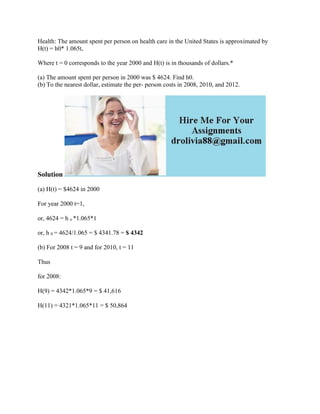Successfully reported this slideshow.

# Health- The amount spent per person on health care in the United State.docx×

# Health- The amount spent per person on health care in the United State.docx

Health: The amount spent per person on health care in the United States is approximated by
H(t) = h0* 1.065t,

Where t = 0 corresponds to the year 2000 and H(t) is in thousands of dollars.*

(a) The amount spent per person in 2000 was \$ 4624. Find h0.
(b) To the nearest dollar, estimate the per- person costs in 2008, 2010, and 2012.
Solution
(a) H(t) = \$4624 in 2000
For year 2000 t=1,
or, 4624 = h o *1.065*1
or, h 0 = 4624/1.065 = \$ 4341.78 = \$ 4342
(b) For 2008 t = 9 and for 2010, t = 11
Thus
for 2008:
H(9) = 4342*1.065*9 = \$ 41,616
H(11) = 4321*1.065*11 = \$ 50,864
.

Health: The amount spent per person on health care in the United States is approximated by
H(t) = h0* 1.065t,

Where t = 0 corresponds to the year 2000 and H(t) is in thousands of dollars.*

(a) The amount spent per person in 2000 was \$ 4624. Find h0.
(b) To the nearest dollar, estimate the per- person costs in 2008, 2010, and 2012.
Solution
(a) H(t) = \$4624 in 2000
For year 2000 t=1,
or, 4624 = h o *1.065*1
or, h 0 = 4624/1.065 = \$ 4341.78 = \$ 4342
(b) For 2008 t = 9 and for 2010, t = 11
Thus
for 2008:
H(9) = 4342*1.065*9 = \$ 41,616
H(11) = 4321*1.065*11 = \$ 50,864
.